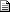# Maths - Rotation about any point - forum discussion

 By: Nobody/Anonymous - nobodyError in 3D rotation around a point matrices   2005-05-26 08:03 On this webpage, I believe I have found an error:    https://www.euclideanspace.com/maths/geometry/affine/aroundPoint/index.htm    It says:    So matrix representing rotation about a given point is:    [R] = [T]-1 * [R0] * [T]    However with the matrices given, you have to use column vectors and calculate the rotated point by;    [New] = [R] * [Old]    You can see by looking at this and the equation for [R] that it will give you the wrong results. In order to make it work, you need to swap the translate and inverse translate matrices, so you would re-write your equation like this:    [R] = [T] * [R0] * [T]-1    And then re-work you matrix products to correct them. Alternatively, you could use row vectors and leave your equation as it is, but transpose your elementary (T, R0, and T-1) matrices and re-work the products.    Regards,  Richard  SirRichard@fascinationsoftware.com
 Richard,    Thank you very much for this, I agree with what you say and I'll update the page.    I think perhaps what I did wrong is after saying that rotate about arbitrary point is equivalent to the following 3 steps:    1) translate the arbitrary point to the origin (which should be translate by -Px,-Py,-Pz)  2) rotate about the origin   3) then translate back (which should be translate by +Px,+Py,+Pz)    Then if the arbitrary point is (Px,Py,Pz) the first translation is -Px,-Py,-Pz, then the rotation, then add +Px, +Py, +Pz.    So if we are using the global frame-of-reference (as explained here: https://www.euclideanspace.com/maths/geometry/rotations/for/  ) then,    [resulting transform] = [third transform] * [second transform] * [first rotation]    [resulting transform] = [+Px,+Py,+Pz] * [rotation] * [-Px,-Py,-Pz]    Which, as you say, inverts the translation component of the resulting transform.    Thanks,    Martin

 metadata block see also: Correspondence about this page Book Shop - Further reading. Where I can, I have put links to Amazon for books that are relevant to the subject, click on the appropriate country flag to get more details of the book or to buy it from them.3D Math Primer - Aimed at complete beginners to vector and matrix algebra. Other Math Books

This site may have errors. Don't use for critical systems.